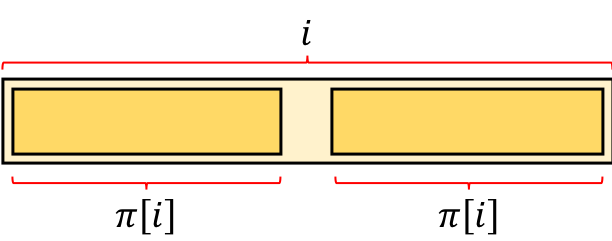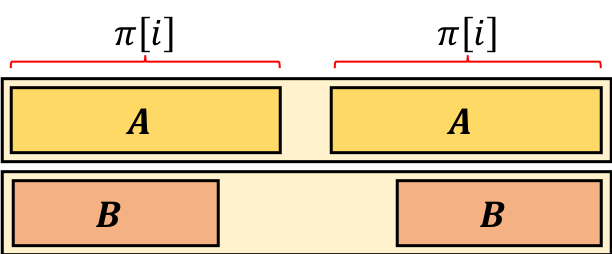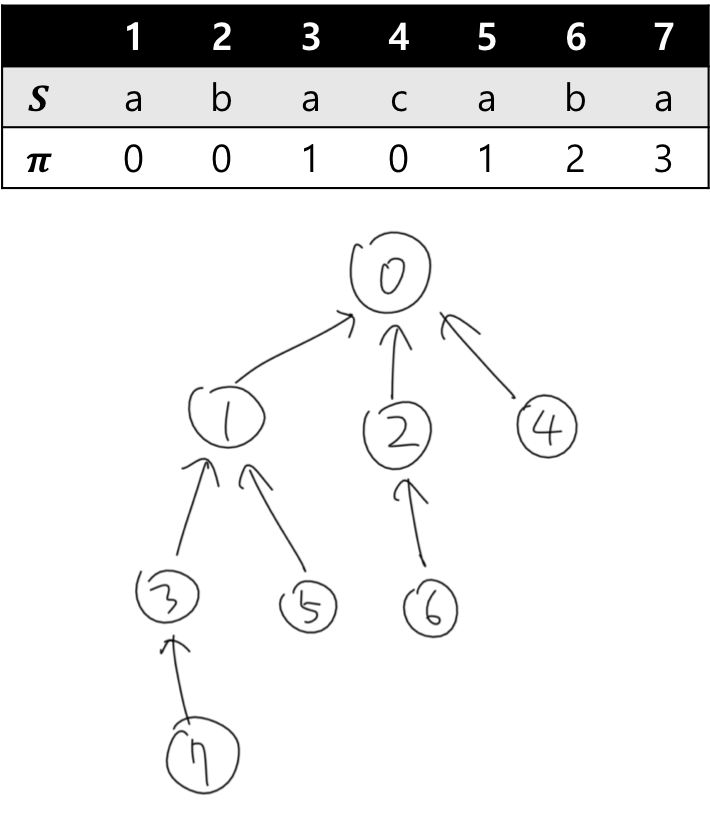# BORDER - Editorial

Tester: Misha Chorniy
Editorialist: Full name

MEDIUM

### PREREQUISITES:

failure function or border array from KMP, binary jumps

### PROBLEM:

Given a string S of length N (\le 200\,000), process Q (\le 200\,000) queries of the form:

• Given i and k (1 \le k \le i \le N), find the length of the k-th shortest border of S[1..i].

### QUICK EXPLANATION:

The length of the borders of S[1..i] are i, \pi[i], \pi[\pi[i]], \pi[\pi[\pi[i]]], \cdots and so on. \pi^{j-1}[i] is the length of the j-th longest border, if it exists. For each i, apply a binary search on j or find the distance from 0 to i, to find \texttt{b}[i], the number of borders of S[1..i]. For each given query, compute the (\texttt{b}[i] - k + 1)-th longest border using binary jumps.

### EXPLANATION:

Let’s start from the most straightforward way. For each query (i, k), consider all prefixes and suffixes in increasing length (there are i of them), and find whether they are the same. (by naive comparision, which needs at most i comparisons) Repeat this process until we find exactly k borders. In pseudocode:

num_borders = 0 // number of borders that we found
for (l = 1 to i) { // l denotes the length of the prefix and the suffix
if (S[1..l] == S[i-l+1..i]) { // if prefix and the suffix are the same
num_borders = num_borders + 1 // then it is a border
if(num_borders == k) { // found k borders!
print l
break
}
}
}
if (num_borders < k) { // there are less than k borders
print -1
}


The time complexity of this algorithm is O(Q \cdot N^2), when there are Q queries and all i = k = N. It is sufficient to solve this subtask, since Q and N are both small.

Here, only N is small and Q can be super-large, which suggests us to precompute some stuff before processing the queries.

For each query (i, k), the problem statement says we are required to

• consider a list of all borders of S[1..i] sorted according to their length
• find the length of k-th one in the list

And that immediately givs us what we should do: For each i, make a sorted list \texttt{L}[i] of all borders of S[1..i] before handling all queries. For each query (i, k), just find the k-th element of the list \texttt{L}[i]. Now, we can handle each query in constant time.

Then, how to make the list \texttt{L}[i]? It is just similar to the algorithm of subtask 1: iterate all i and length l, and append l to the list \texttt{L}[i] if S[1..l] = S[i-l+1..i]. The time complexity of this part is O(N^3) (O(N^2) due to iterating i and l, O(N) due to comparing two strings)

The time complexity of this solution is O(N^3 + Q).

This editorial assumes that you have knowledge on KMP algorithm and know what a “failure function (or border array)” is. If you don’t, try to read these tutorials: topcoder, geeksforgeeks.

After you read the word “border”, or look at the sentence “a border of a string is a substring that appears both as prefix and suffix”, KMP algorithm should pop up in your mind. It is just inside the definition of the failure function (or border array)!

In KMP, \pi[i] is defined as the maximum length of borders of S[1..i] (but except S[1..i] itself). If no such border exists, \pi[i] = 0. By computing this array, we can be sure of the length of two longest borders of S[1..i]: i (itself) and \pi[i] (if \pi[i] > 0).What about other borders? Suppose the third longest border exists, and denote it as B.From the left side of the picture, we can see that B is a prefix of A. From the right side of the picture, we can see that B is a suffix of A. From the definition of B, the length of B should be as long as possible.

Therefore, we can conclude that B is the longest border of A! A equals to S[1..\pi[i]], so the length of B equals to \pi[\pi[i]]. If we apply this logic recursively, we can find that the length of the fourth longest border is \pi[\pi[\pi[i]]], fifth is \pi[\pi[\pi[\pi[i]]]], and so on.

For the sake of simplicity, let’s define \pi^0[i] = i, \pi^{m}[i] = \pi[\pi^{m-1}[i]] for m \ge 1. For example, \pi^{3}[i] = \pi[\pi[\pi[i]]]. We can observe:

• The length of the j-th largest border of S[1..i] is \pi^{j-1}[i].
• For each i, a positive integer m such that \pi^{m}[i] = 0 exists. This is because 0 \le \pi^{j}[i] < \pi^{j-1}[i] (implied from 0 \le \pi[x] < x)
• There are exactly m borders of S[1..i], for such m.
• In conclusion, the lengths of all borders of S[1..i] in increasing order are: \pi^{m-1}[i], \pi^{m-2}[i], \cdots, \pi^{1}[i], \pi^{0}[i].

From these observations, we can solve this problem by:

1. For each i, compute the number of borders \texttt{b}[i] by finding a positive integer m such that \pi^{m}[i] = 0.
2. For each query (i, k), if k \le \texttt{b}[i], print \pi^{\texttt{b}[i]-k}[i], otherwise print -1.

If we do these operations naively, preprocessing part (#1) takes O(N^2) time and processing each query takes O(N) time. (consider the case when all characters are the same) We need more efficient approach.

Note that \pi[x] < x holds for all x. Therefore, we can make a tree structure with n+1 nodes (numbered from 0 to n) where \pi[i] is the parent node of node i.From the picture above, we can see:

1. \texttt{b}[i] equals to the level of node i.
2. For each query (i, k), the answer is the (\texttt{b}[i]-k)-th parent of i (if it exists)

Now it seems more familiar to us! #1 can be done in many ways. (one example is doing a DFS on the tree) #2 can be done efficiently using binary lifting (this is the tutorial, take a look at “Another easy solution in <O(N logN, O(logN)>”).

The idea of it is to compute an additional array \texttt{up}[j,i] which stores the 2^{j}-th parent of node i (in this particular problem, it is \pi^{2^j}[i]) This can be computed as:

\texttt{up}[j, i] = \texttt{up}\Big[j-1, \texttt{up}[j-1, i]\Big]

This is because 2^j = 2^{j-1} + 2^{j-1} holds, so to compute the 2^{j}-th parent of node i one can just go to the 2^{j-1}-th parent of node i and compute its 2^{j-1}-th parent. Since the maximum j is about O(\log N), the time complexity of computing the array \texttt{up} is O(N \log N).

After computing this array, one can find the x-th parent by representing x as a binary number: x = 2^{a_1} + 2^{a_2} + \cdots + 2^{a_l} and find the 2^{a_1}-th parent of 2^{a_2}-th parent of … 2^{a_l}-th parent of i. In pseudocode:

// we want to find the x-th parent of node i
for (a = 20 to 0) { // we find the binary representation from the highest bit to the lowest bit.
if(x >= pow(2, a)) { // in this case, the a-th bit of x is 1.
i = up[a, i] // move 2^a times
x -= pow(2, a)
}
}


It takes O(\log N) time to process each query, since there are at most O(\log N) bits in the binary representation of k.

Therefore, the time complexity of this solution is O(N \log N + Q \log N).

### AUTHOR’S AND TESTER’S SOLUTIONS:

Author’s solution can be found here.
Tester’s solution can be found here.
Second Tester’s solution can be found here.

### RELATED PROBLEMS:

3 Likes

What is border string is still a question to me… please can anyone explain me clearly ?
And in 1st subtask soln from where did string S come from ?? In a border is it compulsory that no. Of characters from prefix should be equal to no. Of characters of suffix ? For example if string is ababab the abab is a border substring… but it’s combination of aba and b or ab and ab or a bab or what ?

@l_returns
A border string is simply a string that appears as both a prefix and suffix of some string.
For example, in the string abcabc, abc is a border string of abcabc since it appears as both a prefix i.e. abcabc and as a suffix abcabc.
And in the string you asked, ababab, abab is a border because it appears as a prefix ababab and as a suffix ababab.

1 Like

Can someone provide a simple solution to this ??

Okay @fayaz_007 thanks…I got that…

A simpler solution is required or a simple explanation to the above editorial will be appreciated